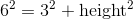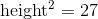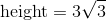# SSAT Upper Level Math : How to find the height of a triangle

## Example Questions

### Example Question #1 : How To Find The Height Of A Triangle

The side of an equilateral triangle, in feet, is. What is the height of this triangle?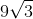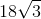Explanation:

The triangle in question looks like this: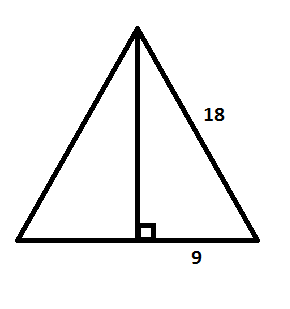The height of a triangle will always cut one of the sides of an equilateral triangle in half. Now, to find the length of the height is just a matter of using the Pythagorean Theorem.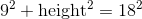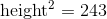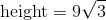### Example Question #2 : How To Find The Height Of A Triangle

The length of a side of an equilateral triangle isfeet. In feet, what is the height of this triangle?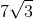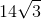Explanation:

The given equilateral should look similar: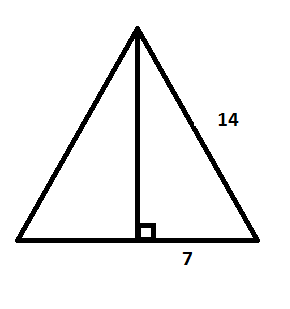Because the height of an equilateral triangle always cuts a side length in half, figuring out the height becomes a matter of applying the Pythagorean Theorem.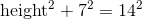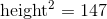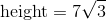### Example Question #3 : How To Find The Height Of A Triangle

The length of a side of an equilateral triangle iscentimeters. In centimeters, what is the length of the height of this triangle?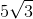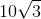Explanation:

Draw out the equilateral triangle: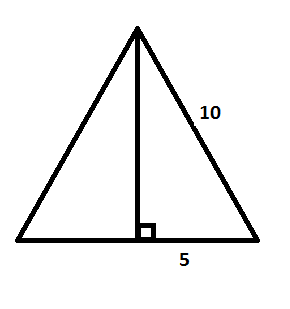Since the height of an equilateral triangle will always cut one of the sides in half, find the height using the Pythagorean Theorem.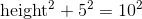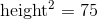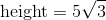### Example Question #4 : How To Find The Height Of A Triangle

The length of a side of an equilateral triangle is. Find the length of the height of this triangle.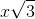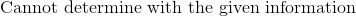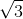Explanation:

Draw out and label the triangle.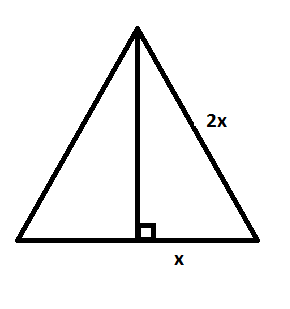Even though you are given exponents for the lengths of this triangle, use the Pythagorean Theorem to solve it. The height of an equilateral triangle will always cut one of its bases in half.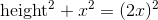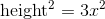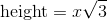### Example Question #5 : How To Find The Height Of A Triangle

Find the height of an equilateral triangle that has side lengths of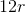.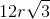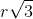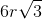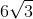Explanation:

Draw out and label the triangle.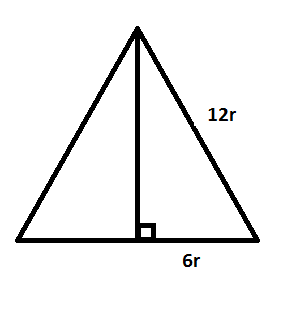Since the height of an equilateral triangle will always cut its base in half, use the Pythagorean Theorem to find the height.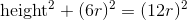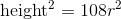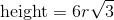### Example Question #6 : How To Find The Height Of A Triangle

Find the height of an equilateral triangle that has a side length of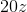.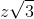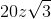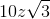Explanation:

Draw and label this triangle: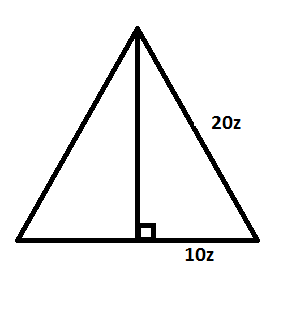Since the height of an equilateral triangle always cuts its base in half, use the Pythagorean Theorem to find the height of the triangle.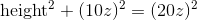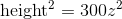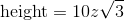### Example Question #7 : How To Find The Height Of A Triangle

Find the height of an equilateral triangle, in yards, that has side lengths ofyards.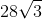Explanation: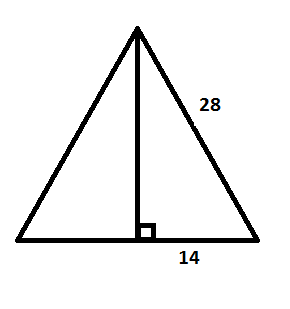Since the height of an equilateral triangle cuts the base in half, use the Pythagorean Theorem to find the height.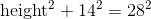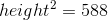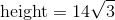### Example Question #8 : How To Find The Height Of A Triangle

An equilateral triangle has sides of length. Find the measure of the height for this triangle.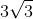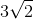Explanation: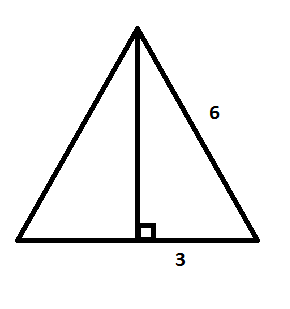The height of an equilateral triangle will always cut the side it intersects in half. Now, this is just a matter of applying the Pythagorean Theorem.